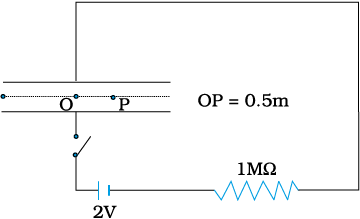A parallel plate capacitor with circular plates of radius 1 m has a capacitance of 1 nF. At t = 0, it is connected for charging in series with a resistor R = 1 M$\Omega$ across a 2V battery (as shown in the figure). Find the magnetic field at a point P, halfway between the centre and the periphery of the plates, after t = 10–3 s. (The charge on the capacitor at time t is (t) = CV[1 – exp (–t/$\tau$)], where the time constant $\tau$ is equal to CR.)Subtopic:  Maxwell's Equations |
To view explanation, please take trial in the course below.
NEET 2023 - Target Batch - Aryan Raj Singh